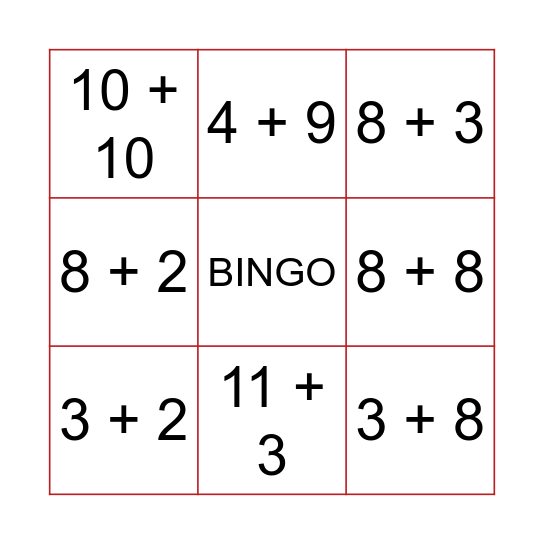This bingo card has a free space and 91 words: 30 + 1, 30+ 2, 30 + 3, 30 + 4, 30 + 5, 14 + 1, 14 + 2, 14 + 3, 14 + 4, 13 + 2, 13 + 3, 15 + 1, 15 + 2, 15 + 3, 11 + 2, 11 + 3, 11 + 4, 11 + 5, 11 + 6, 2 + 7, 2 + 8, 2 + 9, 20 + 2, 20 + 3, 20 + 4, 20 + 5, 10 + 2, 10 + 3, 10 + 4, 10 + 5, 10 + 6, 10 + 7, 10 + 8, 10 + 9, 10 + 10, 8 + 2, 8 + 3, 8 + 4, 8 + 5, 8 + 6, 8 + 7, 8 + 8, 8 + 9, 7 + 2, 7 + 3, 7 + 4, 7 + 5, 7 + 6, 7 + 7, 7 + 8, 7 + 9, 6 + 2, 6 + 3, 6 + 4, 6 + 5, 6 + 6, 6 + 7, 6 + 8, 6 + 9, 5 + 2, 5 + 3, 5 + 4, 5 + 5, 5 + 6, 5 + 7, 5 + 8, 5 + 9, 4 + 2, 4 + 3, 4 + 4, 4 + 5, 4 + 6, 4 + 7, 4 + 8, 4 + 9, 3 + 2, 3 + 3, 3 + 4, 3 + 5, 3 + 6, 3 + 7, 3 + 8, 3 + 9, 2 + 2, 2 + 3, 2 + 4, 2 + 5, 2 + 6, 2 + 7, 2 + 8 and 2 + 9.

⚠ This card has duplicate items: 2 + 7 (2), 2 + 8 (2), 2 + 9 (2)

## Play Online